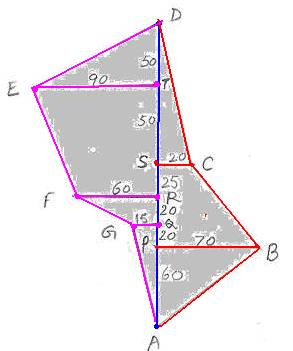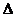6.16 Scale (Surveyors) Drawing.

You must have observed that lands of farmers are not in exact shapes of Triangles or rectangles or trapeziums or parallelograms.

Still, we need to measure the area of lands. Similar is the case when we need to calculate the area of forests or catchments areas of rivers.

Surveyors are the people appointed by Government and other agencies who measure the area of the irregular sized polygon and calculate

their areas for planning purposes. Since fields and rivers have dimensions in kilo meters we use a suitable scale to draw their figures on paper

(For example 1cm = 1meter, 1cm = 100 kilo meters..).

We know how to calculate the areas of:

 No. Object Area Explanation 1 Triangle (1/2)bh B is the base  and h is the height 2 Rectangle lb l is length and b is breadth 3 Trapezium (1/2)(a+b)h a and b are  lengths of parallel sides, h is the height

6.16 Problem 1:  Calculate the area of the below mentioned figure and represent the data in the table as recorded by surveyors

Method:

 Step Procedure 1 Irregular shaped field is divided in to geometrical shaped objects such as triangles, rectangles, and trapezium 5 Use suitable scale to draw figures on sheet of paper 4 Calculate the areas of individual geometrical shaped objects 5 Multiply the figure by the scales to arrive at actual area

The adjoining figure is represented by surveyors as follows

 No. In meters to Points( on left side of AD) In meters to points In meters to Points( on right side of AD) 1 From A to P 60 From P to B 70 2 From Q to G 15 From A to Q 80 3 From R to F 60 From A to R 100 4 From A to S 125 From S to C 20 5 From T to E 90 From A to T 175 6 From A to D 225

Calculation of areas of different figures: (For drawing the figure, we have used a scale: 1cm = 10 Meter)

 No. Areas of As per formula = in Sq meters1PBA =1/2*70*60 2100 2GQA =1/2*15*80 600 3 Trapezium FRGQ =1/2*(60+15)*20 750 4 Trapezium SCPB =1/2*(20+70)*65 2925 5DSC =1/2*20*100 1000 6 Trapezium ETRF =1/2*(90+60)*75 5625 7ETD =1/2*90*50 2250 Total area 15250

6.16 Summary of learning

 No Points learnt 1 Convert Surveyor’s drawing into shapes of triangles, rectangles and trapezium 2 Use suitable scale and formulae to calculate the area.